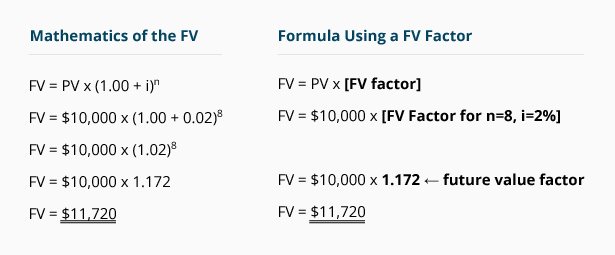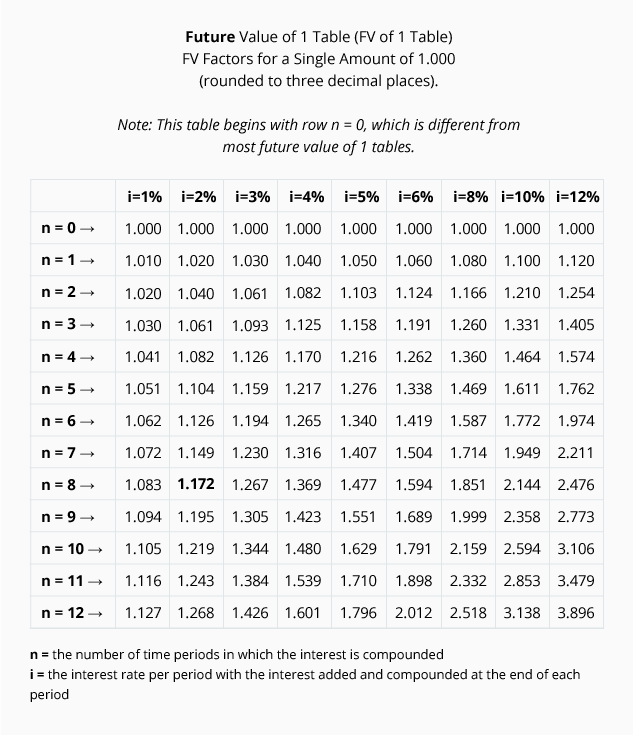# Future Value Factors

The mathematics for calculating the future value of a single amount of \$10,000 earning 8% per year compounded quarterly for two years appears in the left column of the following table. In the right column is the formula which uses a future value factor.Future value factors are available in future value tables, such as the abbreviated version shown here:We highlighted the factor used in our computation. As you can see, the future value factor of 1.172 is located where n = 8, and i = 2%.

Our future value of 1 table is unique in that we have an additional row: n = 0. Most FV of 1 tables omit the row for n = 0, and begin with the row n =1. There should be no difference in FV factors other than minor rounding differences.

To appreciate the usefulness of the FV of 1 table, focus on the column with the heading of i = 10%. This column tells you that the present value of 1.000 is 1.000 at time period 0—the present time. As you move down the 10% column, the next row (where n = 1) shows that the future value will increase by 10% to 1.100. Continuing down the 10% column, you see that at the end of two periods (n = 2) the future value is 1.210, an increase of 0.110 (1.100 x 10%). The next figure down indicates that at the end of three periods the future value is 1.331, which is an increase of 0.121 (1.331 – 1.210; and 1.210 x 10%).

The FV of 1 table provides the future amounts at compound interest for a single amount of 1.000 at various interest rates. These factors should make the future calculations a bit simpler than calculations using exponents.

The 10% column of the future value table can be used to determine the future value of a single \$1.00 invested today at 10% interest compounded annually. The single \$1.00 amount will grow to \$3.138 at the end of 12 years. The FV table also provides some insight as to the future cost of items that are expected to increase at a constant rate. For example, if a cup of coffee presently costs \$1.00 and the cost is expected to increase by 10% per year compounded annually, then a cup of coffee will cost \$3.138 per cup at the end of 12 years.

We can also use the factors for amounts greater than \$1. For example, if the monthly cost of a family's health insurance plan is \$1,000 at the present time and it is expected to increase by 10% per year compounded annually, then the monthly cost at the end of 12 years will be \$3,138.

Throughout the remainder of this topic we will use a more complete Future Value of 1 Table: